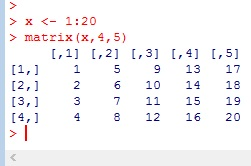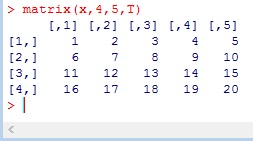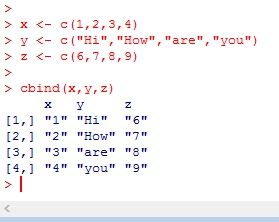# R: Building Matrices

Working with matrices is very useful when working with data. A matrix gives you a two dimensional table similar to what you are used to in programs like Excel. In R, there are couple of different ways in which you can build a matrix.

## Matrix()

The syntax for this command is matrix(data, number of rows, number of columns, fill by row, dimension names)

Lets start slow:

Lets build our data:

x <- 1:20

Now, lets build a 4×5 matrixNotice how the numbers fill in by column, with column 1 being 1-4, column 2 then picks up with 5-8.

If you want to have your numbers fill in by Rows instead, we need to add a fourth argument. We are going to set Fill By Row to True.Now the final argument will be the dimension names. If you want something other than the numbers assigned by default, you need to provide dimension names in the form of a List## Get Data From Matrix

First let us assign the matrix to  a variable. I am using capital “A”. Capital letters are commonly used to represent matrices. You will see this in most Linear Algebra books.

We call information out of the matrix by using the place in the table.

A[row, column]

• A[1,3] – I call the number 3- which is located in the 1rst Row 3rd Column
• A[1,] – returns the entire 1rst row
• A[,1] -returns the entire 1rst column
• A[“Row3″,”C”] – shows you can use the dimension names if you wish## rbind()

rbind() stands for row bind. This is a method for binding multiple vectors into a matrix by row.

First we create 3 vectors (x,y,z) then we turn them in a matrix using rbind()## cbind()

cbind() is just like rbind() but it lines yours vectors up by columns## Change Dimension Names

If you want to change the dimension names after the matrix has already been created.## Code

```x <- 1:20

# build a 4 by 5 matrix
matrix<-(x,4,5)

#fill columns rows first
matrix<-(x,4,5,T)

matrix(x,4,5,T,list(c("Row1","Row2","Row3","Row4"),c("A","B","C","D","E")))

#assign the matrix to a variable
A <- matrix(x,4,5,T,list(c("Row1","Row2","Row3","Row4"),c("A","B","C","D","E")))

#call matrix
A[1,3]

#call row
A[1,]

#call column
A[,1]```
```#call data point by row and column name
A[“Row3”,”C”]

#rbind()
x <- c(1,2,3,4)
y <- c("Hi","How","are","you")
z <- c(6,7,8,9)

rbind(x,y,z)

#cbind()
x <- c(1,2,3,4)
y <- c("Hi","How","are","you")
z <- c(6,7,8,9)

#change dimension names
A <- cbind(x,y,z)
colnames(A) = c("C1","C2","C3")
row.names(A) = c("R1","R2","R3","R4")
A```